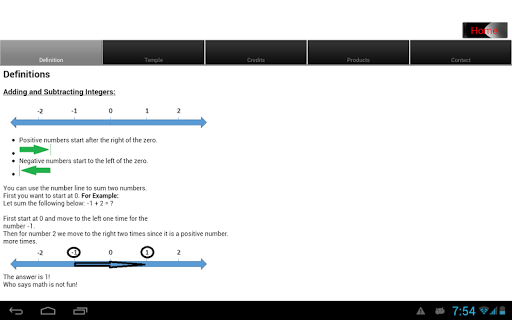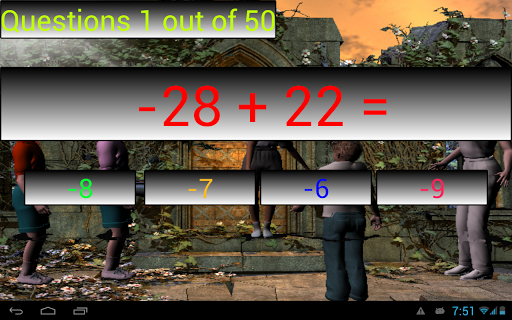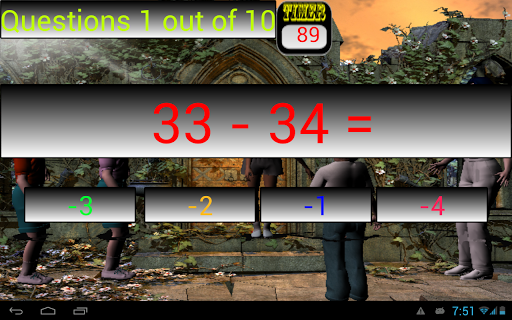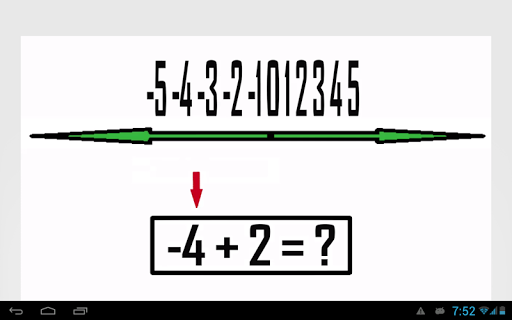Learn to add and subtract positive and negative numbers with this android app. This app includes the following:

1. 50 Practice questions2. 10 timed questions

3. Song on positive and negative numbers by4. Featuring: The Rappin Mathematician with "The Number Line Dance"

5. Step by Step instructions on how to how to Add and Subtract positive and negative integers using the number line.At Education Fun Online, we make education fun! Check weekly for new math apps by Education Fun Online.

Check out other android apps by Education Fun Online:Multiplication String

Reducing FractionsSubtracting Fractions with Like DenominatorsMultiplying Fractions

Dividing FractionsFraction and Whole Number Multiplication Temple Theme

Division Fun FreeDivision Remainder Free

Long DivisionDeterminant 2x2 MatrixMultiplying Decimal Free

Divide DecimalGCF

Prime Numbers